# Nature of the Quadratic Equation, Geometrical Represent - Quadratic Equations, Class 10, Mathematics Notes | Study Additional Documents & Tests for Class 10 - Class 10

## Class 10: Nature of the Quadratic Equation, Geometrical Represent - Quadratic Equations, Class 10, Mathematics Notes | Study Additional Documents & Tests for Class 10 - Class 10

The document Nature of the Quadratic Equation, Geometrical Represent - Quadratic Equations, Class 10, Mathematics Notes | Study Additional Documents & Tests for Class 10 - Class 10 is a part of the Class 10 Course Additional Documents & Tests for Class 10.
All you need of Class 10 at this link: Class 10

Nature of the Roots of The Quadratic Equation

Let the quadratic equation be ax2 + bx + c = 0, ...(i) where a ≠ 0 and a,b,c ∈ R.
The roots of the given equation are given by x =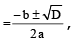where D = b2 – 4ac is the discriminant. i.e., if α and β are two roots of the quadratic equation (i). Then,

α =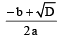and β =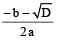Now, the following cases are possible.

Case-I : When D > 0, Roots are real and unequal (distinct).
The roots are given by α =and β =Remark : Consider a quadratic equation ax2 + bx + c = 0, where a,b,c∈Q, a ≠ 0 and D > 0 then :

(i) If D is a perfect square, then roots are rational and unequal.

(ii) If D is not a perfect square, then roots are irrational and unequal. If one root is of the form p + √q (where p is rational and √q is a surd) then the other root will be p – √q
Case-II : When D = 0, Roots are real and equal and each root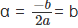Case-III: When D < 0, No real roots exist. Both the roots are imaginary.

Remark : If D < 0, the roots are of the form a ± ib (a,b∈R & i =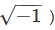. If one root is α + ib, then the other root will be a – ib.
e.g. x2 – 3x + 12 = 0 has D = – 39 < 0

∴ It's roots are, α =and β =or α =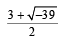and β =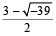or α =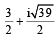and β =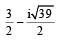COMPETITION WINDOW⇒GEOMETRICAL REPRESENTATION OF QUADRATIC EXPRESSIONConsider the quadratic expression, y = ax2 + bx + c, a ≠ 0 & a,b,c ∈ R then :(i) The graph between x,y is always a parabola. If a > 0, then the shape of the parabola is concave upwards & if a < 0 then the shape of the parabola is concave downwards.(ii) The graph of y = ax2 + bx + c can be divided into 6 categories which are as follows : (Let the roots of the equation ax2 + bx + c = 0 be a and b)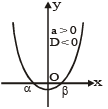Fig(i) Roots are real and distinct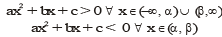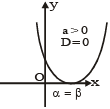Fig(ii) Roots are  coincidentax2 + bx + c > 0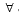x ∈ R–{a }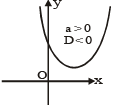Fig(iii) Roots are  complex conjugatesax2 + bx + c > 0x ∈ R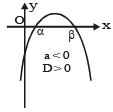Fig(i) Roots are real and distinct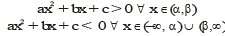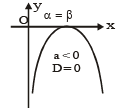Fig(ii) Roots are  coincidentax2 + bx + c < 0x ∈ R–{a }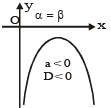Fig(iii) Roots are  complex conjugatesax2 + bx + c < 0x ∈ R Remark : (i) The quadratic expression ax2 + bx + c > 0x ∈ R ⇒ a > 0, D < 0 (fig (iii)) (ii) The quadratic expression ax2 + bx + c < 0x ∈ R ⇒ a < 0, D < 0 (fig (vi))

Ex.9 Find the nature of the roots of the following equations. If the real roots exist, find them.∴
(i) 2x2 – 6x + 3 = 0 (ii) 2x2 – 3x + 5 = 0

Sol. (i) The given equation 2x2 – 6x + 3 = 0
comparing it with ax2 + bx + c = 0, we get a = 2, b = – 6 and c = 3.

∴ Discriminant, D = b2 – 4ac = (–6)2 – 4.2.3 = 36 – 24 = 12 > 0

∵ D > 0, roots are real and unequal.
Now, by quadratic formula, x =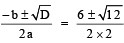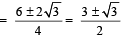Hence the roots are x =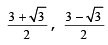(ii) Here, the given equation is 2x– 3x + 5 = 0;
Comparing it with ax+ bx + c = 0, we get a = 2, b = – 3 and c = 5

∴ Discriminant, D = b2 – 4ac = 9 – 4 × 2 × 5 = 9 – 40 = – 31

∵  D < 0, the equation has no real roots.

Ex.10 Find the value of k for each of the following quadratic equations, so that they have real and equal roots:
(i) 9x2 + 8kx + 16 = 0 (ii) (k + 1) x2 – 2(k – 1) x + 1 = 0

Sol. (i) The given equation 9x2 + 8kx + 16 = 0 comparing it with ax2 + bx + c = 0, we get a = 9, b = 8k and c = 16

∴ Discriminant, D = b2 – 4ac = (8k)2 – 4 × 9 × 16 = 64k2 – 576
Since roots are real and equal, so D = 0 ⇒ 64k2 – 576 = 0 ⇒ 64k2 = 576
Hence, k = 3, – 3

(ii) The given equation is (k + 1) x2 – 2(k – 1) x + 1 = 0

comparing it with ax2 + bx + c = 0, we get a = (k + 1), b = – 2 (k – 1) and c = 1
∴ Discriminant, D = b2 – 4ac = 4 (k – 1)2 – 4 (k + 1) × 1
= 4(k2 – 2k + 1) – 4k – 4 ⇒ 4k2 – 8k + 4 – 4k – 4 = 4k2 – 12k

Since roots are real and equal, so D = 0 ⇒ 4k2 – 12k = 0 ⇒ 4k (k – 3) = 0 ⇒ either k = 0 or k – 3 = 0 ⇒ k = 0 or k = 3
Hence, k = 0 , 3.

Ex.11 Find the set of values of k for which the equation kx2 + 2x + 1 has distinct real roots.
Sol. The given equation is kx2 + 2x + 1 = 0

∴ D = (4 – 4 × k × 1) = 4 – 4k.
For distinct and real roots, we must have, D > 0.
Now, D > 0 ⇔ (4 – 4k) > 0 ⇔ 4 > 4k ⇔ 4k < 4 ⇔ k < 1.
∴ Required set = {k ∈ R : k < 1}

Ex.12 Find the values of k for which the equation 5x2 – kx + 4 = 0 has real roots.

Sol. The given equation is 5x2 – kx + 4 = 0∴ D = k2 – 4 × 5 × 4 = k2 – 80
For real roots, we must have, D ≥ 0
Now, D ≥ 0 ⇔ k2 – 80 ≥ 0 ⇔ k2 ≥ 80 ⇔ k ≥ 8 0 or k ≤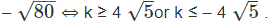COMPETITION WINDOWROOTS  UNDER PARTICULAR CASES (A) Let the quadratic equation ax2 + bx + c = 0 has real roots and(i) If b = 0 ⇔ roots are of equal magnitude but of opposite sign.(ii) If c = 0 ⇔ one root is zero and the other is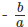(iii) If a = c ⇔ roots are reciprocal of each other.(iv) If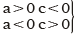⇔ roots are of opposite signs. (v) If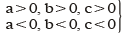⇔ both roots are negative (α + β < 0 & αβ > 0) (vi) If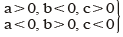⇔ both roots are positive (α + β > 0 & αβ > 0)  (vii) If a + b + c = 0 ⇔ One of the roots is 1 and the other root is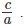(viii) If a = 1, b, c ∈ Z and the roots are rational numbers, then these roots must be integers.(ix) If a, b, c ∈ Q and D is a perfect square ⇔ roots are rational.(x) (A) If a, b, c ∈ Q and D is positive but not a perfect square ⇔ roots are irrational.(B) If ax2 + bx + c = 0 is satisfied by more than two values, it is an identity and a = b = c = 0 and vice versa.(C) The quadratic equation whose roots are reciprocal of the roots of ax2+ bx + c = 0 is cx2 + bx + a = 0 (i.e., the coefficients are written in reverse order).

Sum and Product of The Roots

Let α and β be the roots of the quadratic equation ax+ bx + c = 0, a ≠ 0.
Then α =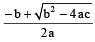and  β =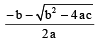∴ The sum of roots α + β =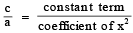and product of roots  = α·β =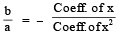Consider the quadratic equation ax+ bx + c = 0, a ≠ 0.
Let α  and β be the roots of the quadratic equation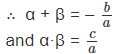Hence the quadratic equation whose roots are α  and β is given by
x2  – (α + β) x + αβ = 0
i.e. x2 – (sum of the roots) x + product of the roots = 0

Ex. 13 Form the quadratic equation in each of the following cases when the roots are : (i) 2 +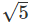and 2 –(ii) a and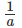Sol. (i) Here roots are a = 2 + 5 and b = 2 – 5
∴ Sum of roots = a + b = (2 + 5 ) + (2 – 5 )
∴ a + b = 4
and product of the roots = a · b = (2 + 5 )·(2 – 5 ) = 4 – 5 = –1
∴ a + b  = – 1
∴  Required equation is x– (sum of roots) x + product of roots = 0 or x2  – (α + β) x + αβ = 0 or x2 – (4) x + (–1) = 0
∴ x2 – 4x – 1 = 0

(ii) Here roots are a and 1/a
∴ α + β = a + 1/a and α . β = a × 1/a  = 1
Here the required equation is x2 –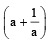x + 1 = 0

 COMPETITION WINDOWCONDITION FOR TWO QUADRATIC EQUATIONS TO HAVE A COMMON ROOTSuppose that the quadratic equations ax2 + bx + c = 0 and a'x2 + b'x + c' = 0 (where a, a' ≠ 0 and ab' – a'b ≠ 0) have a common root. Let this common root be a Then aa2 + ba +c = 0 and a'a2 + b'a +c' = 0 Solving the above equations, we get,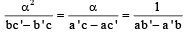⇒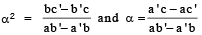Eliminating α , we get :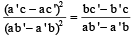⇒ (a'c – ac')2 = (bc' – b'c) (ab' – a'b)This is the required condition for two quadratic equations to have a common root. To obtain the common root, make coefficient of x2 in both the equations same and subtract one equation from the other to obtain a linear equation in x. Solve it for x to obtain the common root.Ex. For which value of k will the equations x2 – kx – 21 = 0 and x2 – 3kx + 35 = 0 have one common root.Sol. Let the common root be a, then, α 2 - kα  - 21 = 0 and α 2 - 3kα  + 35 = 0.Solving by cramer rule, we have :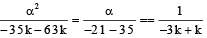∴ α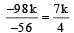and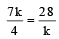⇒ 7k2 = 28 × 4 ⇒ k = ± 4CONDITION FOR TWO QUADRATIC EQUATIONS TO HAVE THE SAME ROOTSTwo quadratic equations ax2 + bx + c = 0 and a'x2 + b'x + c' = 0 have the same roots if and only if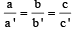Algorithm : The method of problem solving consists of the following three steps :–

Step-I : Translating the word problem in to symbolic language (mathematical statement) which means identifying relationships existing in the problem & then forming the quadratic equation.

Step-II : Solving the quadratic equation thus formed

Step-III : Interpreting the solution of the equation which means translating the result of mathematical statement into verbal language.

Type-I : Problems Based On Numbers.

Ex.14 The difference of two numbers is 3 and their product is 504. Find the numbers.

Sol. Let the required numbers be x and (x – 3).
Then, x ( x – 3) = 504 ⇒ x2 – 3x – 504 = 0
⇒ x2 – 24x + 21x – 504 = 0 ⇒ x (x – 24) + 21 (x – 24) = 0
⇒ (x – 24) (x + 21) = 0 ⇒ x – 24 = 0 or x + 21 = 0
⇒ x = 24 or x = – 21
If x = – 21, then the numbers are – 21 and – 24.
Again, if x = 24, then the numbers are 24 and 21.
Hence, the numbers are – 21, – 24 or 24, 21

Ex.15 The sum of the squares of two consecutive odd positive integers is 290. Find the integers.

Sol. Let the two consecutive odd positive integers be x and (x + 2). Then, x2 + (x + 2)2 = 290
⇒ x2 + x2 + 4x + 4 = 290
⇒ x+ 2x – 143 = 0 ⇒ x2 + 13x – 11x – 143 = 0
⇒ x (x + 13) – 11 (x + 13) = 0 ⇒ (x + 13) (x – 11) = 0
⇒ x = – 13 or x = 11 But – 13, is not an odd positive integer.
Hence, the required integers are 11 and 13.

Type-II : Problems Based On Ages :

Ex.16 Seven years ago Varun's age was five times the square of Swati's age. Three years hence, Swati's age will be two fifth of Varun's age. Find their present ages.

Sol. Let the present ages of Varun and Swati be x years and y years respectively.
Seven years ago,
Varun's age = (x – 7) years and Swati's age  = (y – 7) years.
∴ (x  – 7) = 5 (y – 7)2 ⇒ x – 7 = 5 (y2 – 14 y + 49)
⇒ x = 5y2 – 70y + 245 + 7 ⇒ x = 5y– 70y + 252           ...(i)
Three years hence,
Varun's age = (x + 3) years and Swati's age = (y + 3) years.
∴ (y + 3) = 2/5
(x + 3) ⇒ 5y + 15 = 2x + 6 ⇒ x =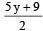...(ii)
From (i) and (ii) we get 5y2 – 70y + 252 =⇒ 10y2 – 140y + 504 = 5y + 9    ⇒ 10y2 – 145y + 495 = 0 ⇒ 2y– 29y + 99 = 0 ⇒ 2y– 18y – 11y + 99 = 0 ⇒ 2y (y – 9) – 11 (y – 9) = 0

⇒ (y – 9) (2y – 11) = 0 ⇒ y = 9 or y = 11/2
∵ y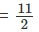is not possible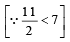So, y = 9.
∴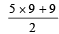= 27 [From (ii)]

Hence, the Varun's present age is 27 years and Swati's present age is 9 years.

Type-III : Problems Based On Geometrical Concepts :

Ex.17 The length of the hypotenuse of a right triangle exceeds the length of the base by 2 cm and exceeds twice the length of the altitude by 1 cm. Find the length of each side of the triangle.

Sol. Let DABC be a right triangle, right angled at B.
Let AB = x. Then AC = (2x + 1) and BC = (2x + 1) – 2 = 2x – 1
⇒ ΔABC, AC= AB+ BC2 [By pythagoras theorem]
⇒ (2x + 1)2 = x2 + (2x – 1)2 ⇒ 4x2 + 4x + 1 = x+ 4x2 – 4x + 1
⇒ x2 = 8x ⇒ x = 8 cm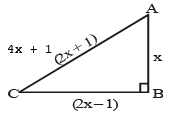∴ BC = 2x – 1 = 2 ×  8 – 1 = 15 cm
AC = 2x + 1= 2 × 8  + 1 = 17cm
Hence, the sides of the given triangle are 8cm, 15 cm and 17 cm.

Type-IV : Problems Based On Perimeter/Area :

Ex.18 Is it possible to design a rectangular park of perimeter 80 cm and area 400 m2? If so, find its length and breadth.

Sol. Let the length and breadth of the rectangular park be l and b respectively.

Then, 2 (ℓ+ b) = 80 ℓ + b = 40 ⇒ ℓ = (40 – b) And area of the park = 400 m2

∴ ℓb = 400 ⇒ (40 – b) b = 400 ⇒ 40b – b2 = 400 ⇒ b2 – 40b + 400 = 0
⇒ b2 – 20b – 20b + 400 = 0 ⇒ b (b – 20) – 20 (b – 20) = 0
⇒ (b – 20) (b – 20) = 0 ⇒ (b – 20)= 0
⇒ b – 20 = 0 ⇒ b = 20 m
∴ ℓ =  40 – b = 40 – 20 = 20 m Hence, length and breadth of the park are 20 m and 20 m respectively.
Thus, It is possible to design a rectangular park of perimeter 80 m and area 400 m2

Type-V : Problems Based On Time and Distance

Ex.19 A train travels 360 km at a uniform speed. If the speed had been 5 km/h more, it would have taken 1 hour less for the same journey. Find the speed of the train.

Sol. Let the speed of the train be x km/h. Then, Time taken to cover the distance of 360 km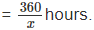If the speed of the train increased by 5 km/h. Then, Time taken to cover the same distance =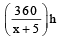According to the question,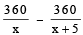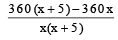⇒ 360x + 1800 – 360x = x2 + 5x ⇒ x2 + 5x – 1800 = 0
⇒ x2 + 45x – 40x – 1800 = 0 ⇒ x (x + 45) –  40 (x + 45) = 0
⇒ (x + 45) (x – 40) = 0 ⇒ x = – 45 or x = 40
But the speed can not be negative.
Hence, the speed of the train is 40 km/h.

Type-VI : Problems Based On Time and Work : :

Ex.20 Two water taps together can fill a tank in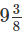hours. The tap of larger diameter takes 10 hours less thanthe smaller one to fill the tank respectively. Find the time in which each tap can separately fill the tank.
Sol. Let the tap of larger diameter takes x hours to fill the tank. Then, the tap of smaller diameter takes (x + 10) hours to fill the tank.

∴ The portion of tank filled by the larger tap in one hour = 1/x ,

the portion of tank filled by the smaller tap in one hour =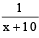And the portion of tank filled by both the smaller and the larger tap in one hour =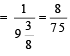⇒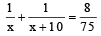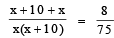⇒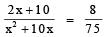⇒ 150x + 750 = 8x2 + 80x ⇒ 8x2 – 70x – 750 = 0
⇒ 4x2 – 35x – 375 = 0 ⇒ 4x2 – 60x + 25x – 375 = 0
⇒ 4x (x – 15) + 25 (x – 15) = 0 ⇒ (x – 15) (4x + 25) = 0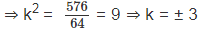⇒ x = 15 or x =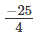but the time can not be negative.
Hence, the larger tap takes 15 hours and the smaller tap takes 25 hours.

Type-VII : Miscellaneous Problems :

Ex.21 300 apples are distributed equally among a certain  number of students.Had there been 10 more students, each would have received one apple less. Find the number of students.
Sol. Let the number of students be x. Then, the number of apples received by each student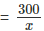If there is 10 more students, i.e., (x + 10) students. Then,

the number of apples received by each student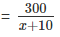According to the question,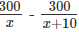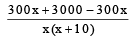⇒ 3000 = x2 + 10x
⇒ x2 + 10x – 3000 = 0
⇒ x2 + 60x – 50x – 3000 = 0
⇒ x (x + 60) – 50 (x + 60) = 0 ⇒ (x + 60) (x – 50) = 0

⇒ x = – 60 or x = 50
But the number of students can not be negative.

Hence, the number of students is 50.

The document Nature of the Quadratic Equation, Geometrical Represent - Quadratic Equations, Class 10, Mathematics Notes | Study Additional Documents & Tests for Class 10 - Class 10 is a part of the Class 10 Course Additional Documents & Tests for Class 10.
All you need of Class 10 at this link: Class 10Use Code STAYHOME200 and get INR 200 additional OFF

## Additional Documents & Tests for Class 10

5 videos|196 docs|42 tests

Track your progress, build streaks, highlight & save important lessons and more!

,

,

,

,

,

,

,

,

,

,

,

,

,

,

,

,

,

,

,

,

,

,

,

,

,

,

,

,

,

,

;